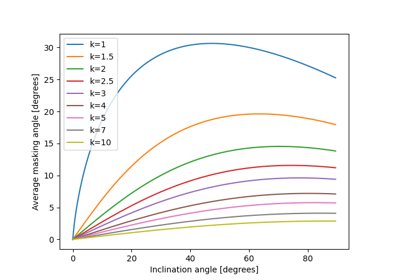The average masking angle over the slant height of a row.

The masking angle is the angle from horizontal where the sky dome is blocked by the row in front. The masking angle is larger near the lower edge of a row than near the upper edge. This function calculates the average masking angle as described in 1.

Parameters
• surface_tilt (numeric) – Panel tilt from horizontal [degrees].

• gcr (float) – The ground coverage ratio of the array [unitless].

Returns

mask_angle (numeric) – Average angle from horizontal where diffuse light is blocked by the preceding row [degrees].

Notes

The pvlib-python authors believe that Eqn. 9 in 1 is incorrect. Here we use an independent equation. First, Eqn. 8 is non-dimensionalized (recasting in terms of GCR):

$\psi(z') = \arctan \left [ \frac{(1 - z') \sin \beta} {\mathrm{GCR}^{-1} + (z' - 1) \cos \beta} \right ]$

Where $$GCR = B/C$$ and $$z' = z/B$$. The average masking angle $$\overline{\psi} = \int_0^1 \psi(z') \mathrm{d}z'$$ is then evaluated symbolically using Maxima (using $$X = 1/\mathrm{GCR}$$):

load(scifac)    /* for the gcfac function */
assume(X>0, cos(beta)>0, cos(beta)-X<0);   /* X is 1/GCR */
gcfac(integrate(atan((1-z)*sin(beta)/(X+(z-1)*cos(beta))), z, 0, 1))


This yields the equation implemented by this function:

$\begin{split}\overline{\psi} = \ &-\frac{X}{2} \sin\beta \log | 2 X \cos\beta - (X^2 + 1)| \\ &+ (X \cos\beta - 1) \arctan \frac{X \cos\beta - 1}{X \sin\beta} \\ &+ (1 - X \cos\beta) \arctan \frac{\cos\beta}{\sin\beta} \\ &+ X \log X \sin\beta\end{split}$

The pvlib-python authors have validated this equation against numerical integration of $$\overline{\psi} = \int_0^1 \psi(z') \mathrm{d}z'$$.

References

1(1,2)

D. Passias and B. Källbäck, “Shading effects in rows of solar cell panels”, Solar Cells, Volume 11, Pages 281-291. 1984. DOI: 10.1016/0379-6787(84)90017-6

## Examples using pvlib.shading.masking_angle_passias#